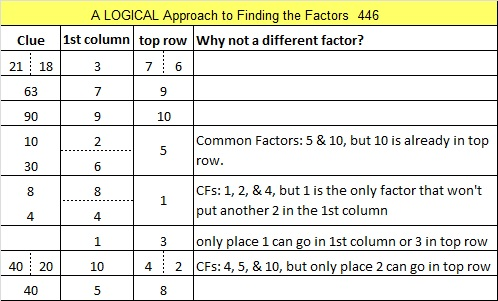# Eight Ways to Make 446 with Three Squares

When I read that 446 is the smallest number that can be expressed as the sum of three squares eight different ways, I just had to find those eight ways for myself. I found them, and it was fun. I made a graphic showing the eight ways:Print the puzzles or type the factors on this excel file: 10 Factors 2015-03-30

• 446 is a composite number.
• Prime factorization: 446 = 2 x 223
• The exponents in the prime factorization are 1 and 1. Adding one to each and multiplying we get (1 + 1)(1 + 1) = 2 x 2 = 4. Therefore 446 has exactly 4 factors.
• Factors of 446: 1, 2, 223, 446
• Factor pairs: 446 = 1 x 446 or 2 x 223
• 446 has no square factors that allow its square root to be simplified. √446 ≈ 21.1187